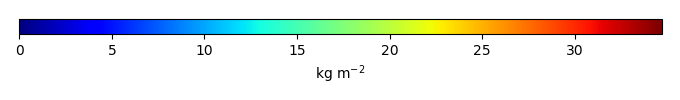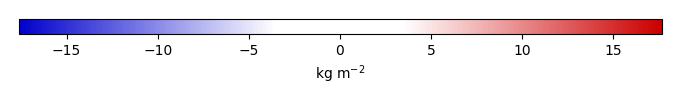# Mean State

Period Mean (original grids) [Pg]
Model Period Mean (intersection) [Pg]
Model Period Mean (complement) [Pg]
Benchmark Period Mean (intersection) [Pg]
Benchmark Period Mean (complement) [Pg]
Bias [kg m-2]
Bias Score 
Spatial Distribution Score 
Overall Score 
Benchmark [-] 64.0
CLM5PHSOFF [-] 47.0 46.0 1.24 63.9 0.0663 -5.30 0.691 0.767 0.729
CLM5PHSON [-] 38.4 38.4 0.429 63.9 0.0663 -7.15 0.634 0.580 0.607
Period Mean (original grids) [Pg]
Model Period Mean (intersection) [Pg]
Model Period Mean (complement) [Pg]
Benchmark Period Mean (intersection) [Pg]
Benchmark Period Mean (complement) [Pg]
Bias [kg m-2]
Bias Score 
Spatial Distribution Score 
Overall Score 
Benchmark [-] 3.34
CLM5PHSOFF [-] 2.39 1.45 0.643 3.34 0.00700 -0.601 0.480 0.548 0.514
CLM5PHSON [-] 3.30 2.21 0.738 3.34 0.00700 -0.331 0.497 0.570 0.533
Period Mean (original grids) [Pg]
Model Period Mean (intersection) [Pg]
Model Period Mean (complement) [Pg]
Benchmark Period Mean (intersection) [Pg]
Benchmark Period Mean (complement) [Pg]
Bias [kg m-2]
Bias Score 
Spatial Distribution Score 
Overall Score 
Benchmark [-] 14.1
CLM5PHSOFF [-] 10.4 8.13 1.85 14.1 0.0194 -0.876 0.483 0.751 0.617
CLM5PHSON [-] 12.3 11.1 0.996 14.1 0.0194 -0.223 0.547 0.791 0.669
Period Mean (original grids) [Pg]
Model Period Mean (intersection) [Pg]
Model Period Mean (complement) [Pg]
Benchmark Period Mean (intersection) [Pg]
Benchmark Period Mean (complement) [Pg]
Bias [kg m-2]
Bias Score 
Spatial Distribution Score 
Overall Score 
Benchmark [-] 1.22
CLM5PHSOFF [-] 3.50 2.66 0.855 1.22 0.00453 0.572 0.513 0.678 0.596
CLM5PHSON [-] 4.18 3.37 0.817 1.22 0.00453 0.991 0.469 0.503 0.486
Period Mean (original grids) [Pg]
Model Period Mean (intersection) [Pg]
Model Period Mean (complement) [Pg]
Benchmark Period Mean (intersection) [Pg]
Benchmark Period Mean (complement) [Pg]
Bias [kg m-2]
Bias Score 
Spatial Distribution Score 
Overall Score 
Benchmark [-] 47.3
CLM5PHSOFF [-] 78.7 67.4 9.09 45.9 1.37 6.09 0.709 0.700 0.705
CLM5PHSON [-] 40.1 38.1 1.81 45.9 1.37 2.33 0.683 0.514 0.598
Period Mean (original grids) [Pg]
Model Period Mean (intersection) [Pg]
Model Period Mean (complement) [Pg]
Benchmark Period Mean (intersection) [Pg]
Benchmark Period Mean (complement) [Pg]
Bias [kg m-2]
Bias Score 
Spatial Distribution Score 
Overall Score 
Benchmark [-] 41.6
CLM5PHSOFF [-] 32.6 31.5 0.606 41.6 0.0303 -1.13 0.506 0.705 0.606
CLM5PHSON [-] 52.3 51.1 0.450 41.6 0.0303 1.23 0.552 0.742 0.647
Period Mean (original grids) [Pg]
Model Period Mean (intersection) [Pg]
Model Period Mean (complement) [Pg]
Benchmark Period Mean (intersection) [Pg]
Benchmark Period Mean (complement) [Pg]
Bias [kg m-2]
Bias Score 
Spatial Distribution Score 
Overall Score 
Benchmark [-] 15.6
CLM5PHSOFF [-] 27.8 23.5 4.20 15.6 0.0349 0.837 0.478 0.660 0.569
CLM5PHSON [-] 31.8 29.6 2.96 15.6 0.0349 1.93 0.506 0.676 0.591
Period Mean (original grids) [Pg]
Model Period Mean (intersection) [Pg]
Model Period Mean (complement) [Pg]
Benchmark Period Mean (intersection) [Pg]
Benchmark Period Mean (complement) [Pg]
Bias [kg m-2]
Bias Score 
Spatial Distribution Score 
Overall Score 
Benchmark [-] 14.8
CLM5PHSOFF [-] 33.0 27.3 3.92 14.6 0.136 2.09 0.481 0.631 0.556
CLM5PHSON [-] 24.9 22.6 1.43 14.6 0.136 2.37 0.529 0.734 0.632
Period Mean (original grids) [Pg]
Model Period Mean (intersection) [Pg]
Model Period Mean (complement) [Pg]
Benchmark Period Mean (intersection) [Pg]
Benchmark Period Mean (complement) [Pg]
Bias [kg m-2]
Bias Score 
Spatial Distribution Score 
Overall Score 
Benchmark [-] 1.78
CLM5PHSOFF [-] 5.64 4.17 1.82 1.78 1.19 0.476 0.670 0.573
CLM5PHSON [-] 6.90 5.31 1.98 1.78 1.79 0.402 0.575 0.489
Period Mean (original grids) [Pg]
Model Period Mean (intersection) [Pg]
Model Period Mean (complement) [Pg]
Benchmark Period Mean (intersection) [Pg]
Benchmark Period Mean (complement) [Pg]
Bias [kg m-2]
Bias Score 
Spatial Distribution Score 
Overall Score 
Benchmark [-] 15.3
CLM5PHSOFF [-] 18.5 13.5 4.37 14.8 0.505 -0.314 0.528 0.811 0.670
CLM5PHSON [-] 11.3 9.25 1.86 14.8 0.505 -0.174 0.552 0.810 0.681
Period Mean (original grids) [Pg]
Model Period Mean (intersection) [Pg]
Model Period Mean (complement) [Pg]
Benchmark Period Mean (intersection) [Pg]
Benchmark Period Mean (complement) [Pg]
Bias [kg m-2]
Bias Score 
Spatial Distribution Score 
Overall Score 
Benchmark [-] 13.1
CLM5PHSOFF [-] 21.3 17.6 3.17 13.1 0.0236 0.832 0.463 0.684 0.574
CLM5PHSON [-] 18.2 17.0 0.899 13.1 0.0236 1.50 0.498 0.694 0.596
Period Mean (original grids) [Pg]
Model Period Mean (intersection) [Pg]
Model Period Mean (complement) [Pg]
Benchmark Period Mean (intersection) [Pg]
Benchmark Period Mean (complement) [Pg]
Bias [kg m-2]
Bias Score 
Spatial Distribution Score 
Overall Score 
Benchmark [-] 455.
CLM5PHSOFF [-] 543. 484. 59.8 449. 5.38 0.329 0.637 0.889 0.763
CLM5PHSON [-] 487. 464. 23.3 449. 5.38 0.776 0.637 0.859 0.748
Period Mean (original grids) [Pg]
Model Period Mean (intersection) [Pg]
Model Period Mean (complement) [Pg]
Benchmark Period Mean (intersection) [Pg]
Benchmark Period Mean (complement) [Pg]
Bias [kg m-2]
Bias Score 
Spatial Distribution Score 
Overall Score 
Benchmark [-] 0.854
CLM5PHSOFF [-] 0.970 0.441 0.430 0.849 0.00531 -0.259 0.422 0.664 0.543
CLM5PHSON [-] 1.51 0.664 0.856 0.849 0.00531 0.0462 0.484 0.722 0.603
Period Mean (original grids) [Pg]
Model Period Mean (intersection) [Pg]
Model Period Mean (complement) [Pg]
Benchmark Period Mean (intersection) [Pg]
Benchmark Period Mean (complement) [Pg]
Bias [kg m-2]
Bias Score 
Spatial Distribution Score 
Overall Score 
Benchmark [-] 2.82
CLM5PHSOFF [-] 11.6 9.02 2.37 2.82 0.00299 1.89 0.374 0.353 0.363
CLM5PHSON [-] 14.5 12.3 2.08 2.82 0.00299 3.01 0.268 0.346 0.307
Period Mean (original grids) [Pg]
Model Period Mean (intersection) [Pg]
Model Period Mean (complement) [Pg]
Benchmark Period Mean (intersection) [Pg]
Benchmark Period Mean (complement) [Pg]
Bias [kg m-2]
Bias Score 
Spatial Distribution Score 
Overall Score 
Benchmark [-] 4.95
CLM5PHSOFF [-] 16.3 13.3 2.28 4.88 0.0615 3.12 0.379 0.398 0.388
CLM5PHSON [-] 10.2 9.16 0.789 4.88 0.0615 3.11 0.449 0.515 0.482
Period Mean (original grids) [Pg]
Model Period Mean (intersection) [Pg]
Model Period Mean (complement) [Pg]
Benchmark Period Mean (intersection) [Pg]
Benchmark Period Mean (complement) [Pg]
Bias [kg m-2]
Bias Score 
Spatial Distribution Score 
Overall Score 
Benchmark [-] 13.0
CLM5PHSOFF [-] 7.07 5.61 1.10 13.0 0.00854 -1.84 0.483 0.627 0.555
CLM5PHSON [-] 11.8 11.4 0.332 13.0 0.00854 -0.100 0.652 0.874 0.763
Period Mean (original grids) [Pg]
Model Period Mean (intersection) [Pg]
Model Period Mean (complement) [Pg]
Benchmark Period Mean (intersection) [Pg]
Benchmark Period Mean (complement) [Pg]
Bias [kg m-2]
Bias Score 
Spatial Distribution Score 
Overall Score 
Benchmark [-] 21.4
CLM5PHSOFF [-] 32.7 31.6 1.13 21.3 0.128 1.87 0.475 0.693 0.584
CLM5PHSON [-] 43.8 43.5 0.471 21.3 0.128 4.72 0.474 0.788 0.631
Period Mean (original grids) [Pg]
Model Period Mean (intersection) [Pg]
Model Period Mean (complement) [Pg]
Benchmark Period Mean (intersection) [Pg]
Benchmark Period Mean (complement) [Pg]
Bias [kg m-2]
Bias Score 
Spatial Distribution Score 
Overall Score 
Benchmark [-] 9.57
CLM5PHSOFF [-] 1.47 1.24 0.324 9.53 0.0397 -2.59 0.402 0.256 0.329
CLM5PHSON [-] 3.09 2.90 0.233 9.53 0.0397 -1.92 0.486 0.493 0.489
Period Mean (original grids) [Pg]
Model Period Mean (intersection) [Pg]
Model Period Mean (complement) [Pg]
Benchmark Period Mean (intersection) [Pg]
Benchmark Period Mean (complement) [Pg]
Bias [kg m-2]
Bias Score 
Spatial Distribution Score 
Overall Score 
Benchmark [-] 151.
CLM5PHSOFF [-] 150. 147. 2.37 151. 0.0403 -0.581 0.784 0.935 0.859
CLM5PHSON [-] 129. 128. 0.570 151. 0.0403 -2.41 0.765 0.777 0.771
Period Mean (original grids) [Pg]
Model Period Mean (intersection) [Pg]
Model Period Mean (complement) [Pg]
Benchmark Period Mean (intersection) [Pg]
Benchmark Period Mean (complement) [Pg]
Bias [kg m-2]
Bias Score 
Spatial Distribution Score 
Overall Score 
Benchmark [-] 10.7
CLM5PHSOFF [-] 20.3 19.1 1.41 10.7 0.0170 1.19 0.509 0.777 0.643
CLM5PHSON [-] 21.5 21.4 0.512 10.7 0.0170 1.83 0.517 0.807 0.662
Period Mean (original grids) [Pg]
Model Period Mean (intersection) [Pg]
Model Period Mean (complement) [Pg]
Benchmark Period Mean (intersection) [Pg]
Benchmark Period Mean (complement) [Pg]
Bias [kg m-2]
Bias Score 
Spatial Distribution Score 
Overall Score 
Benchmark [-] 4.41
CLM5PHSOFF [-] 8.10 7.47 0.837 4.31 0.102 1.33 0.564 0.762 0.663
CLM5PHSON [-] 6.25 5.78 0.493 4.31 0.102 1.41 0.590 0.801 0.695

# Temporally integrated period mean

BENCHMARK MEANMODEL MEANBIASBIAS SCORESPATIAL TAYLOR DIAGRAMMODEL COLORS# Spatially integrated regional mean

MODEL COLORS# All Models

BenchmarkCLM5PHSOFFCLM5PHSON# Data Information

history: Mon Mar 21 09:05:59 2016: ncatted -a units,biomass,o,c,kg m-2 ./from_MQs_site/DATA/biomass/GEOCARBON/derived/biomass_0.5x0.5.nc tmp.nc